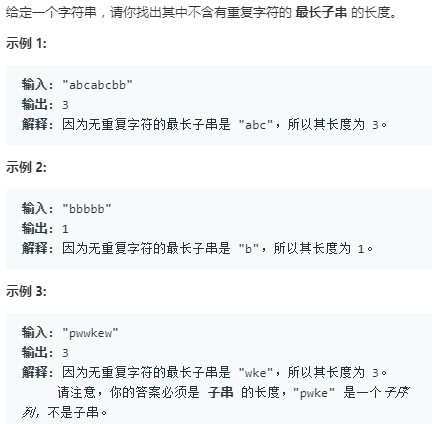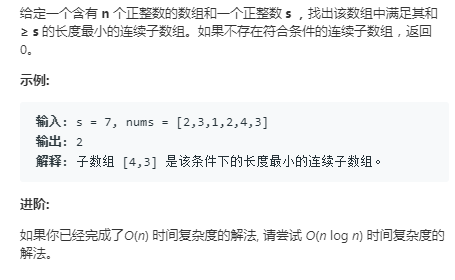• 滑动窗口python

千次阅读 2020-02-04 22:41:05
通过一个滑动窗口来进行比较，当下一个元素与窗口中的元素没有重复时，则推动窗口右边界，使窗口包含该元素，如果下一个元素与窗口中的元素有重复，则推动左边界，使窗口缩小不包含重复的元素，然后右窗口又向右移动...

本专题将讲解的题目为leetcode中的第3题和第209题

【题目】分析：

通过一个滑动窗口来进行比较，当下一个元素与窗口中的元素没有重复时，则推动窗口右边界，使窗口包含该元素，如果下一个元素与窗口中的元素有重复，则推动左边界，使窗口缩小不包含重复的元素，然后右窗口又向右移动继续包含其他元素。每次移动后，我们都记录当前窗口的大小，最后选一个最长的窗口即可。

方法：

1）首先初始化窗口

滑动窗口包含左右两个，因此初始化条件可为left, right = 0, -1

2）终止条件

由于右窗口会先滑到右端，因此终止条件是左窗口在数组长度范围内即可

3）移动条件

由于初始化right为-1，因此当s[right+1]不在数组s（更新的）内，并且长度小于数组的长度，即向右窗口移动，否则向左窗口移动

class Solution:
def lengthOfLongestSubstring(self, s: str) -> int:
left, right = 0, -1  # [left...right]

max_len = 0

# 终止条件
while left < len(s):
# 窗口滑动条件
if right + 1 < len(s) and s[right + 1] not in s[left:right + 1]:
right += 1
else:
left += 1

max_len = max(max_len, right - left + 1)

return max_len

【题目】分析：

跟上一题一样，同样采用滑动窗口，这里直接附上代码

class Solution:
def minSubArrayLen(self, s: int, nums: List[int]) -> int:
#初始化
left, right = 0, -1

# 辅助变量
window_sum = 0
min_len = float("inf")

# 终止条件
while left < len(nums):
# 滑动条件
if window_sum < s and right + 1 < len(nums):
window_sum += nums[right + 1]
right += 1
else:
window_sum -= nums[left]
left += 1
if window_sum >= s:
min_len = min(min_len, right - left + 1) if min_len !=float("inf") else right - left + 1
return min_len if min_len !=float("inf") else 0

展开全文• 滑动窗口 滑动窗口就是能够根据指定的单位长度来框住时间序列，从而计算框内的统计指标。相当于一个长度指定的滑块正在刻度尺上面滑动，每滑动一个单位即可反馈滑块内的数据。 滑动窗口的意义 为了提升数据的准确性...
• 题目 输入：从控制台获取n,m,a,b;... 不了解滑动窗口的可以参考一维滑动窗口这篇文章 源码 from pip._vendor.distlib.compat import raw_input class Solution: #获得输入参数 def get_parameters
• Python滑动平均算法（Moving Average）方案： #!/usr/bin/env python # -*- coding: utf-8 -*- import numpy as np # 等同于MATLAB中的smooth函数，但是平滑窗口必须为奇数。 # yy = smooth(y) smooths the data ...
• So, I am interested in any hints for implementing this flexible sliding window (in Python) without writing and specifying separately each possible situation. To recap: Example of input: ["w1", "w2", ...Problem description: I'm interested in looking at terms in the text window of, say, 3 words to the left and 3 to the right. The base case has the form of w-3 w-2 w-1 term w+1 w+2 w+3. I want to implement a sliding window over my text with which I will be able to record the context words of each term. So, every word is once treated as a term, but when the window moves, it becomes a context word, etc. However, when the term is the 1st word in line, there are no context words on the left (t w+1 w+2 w+3), when it's the 2nd word in line, there's only one context word on the left, and so on. So, I am interested in any hints for implementing this flexible sliding window (in Python) without writing and specifying separately each possible situation.

To recap:

Example of input:

["w1", "w2", "w3", "w4", "w5", "w6", "w7", "w8", "w9", "w10"]

Output:

t1 w2 w3 w4

w1 t2 w3 w4 w5

w1 w2 t3 w4 w5 w6

w1 w2 w3 t4 w5 w6 w7

__ w2 w3 w4 t5 w6 w7 w8

__ __ etc.

My current plan is to implement this with a separate condition for each line in the output.

解决方案

If you want a sliding window of n words, use a double-ended queue with maximum length n to implement a buffer.

This should illustrate the concept:

mystr = "StackOverflow"

from collections import deque

window = deque(maxlen=5)

for char in mystr:

window.append(char)

print ( ''.join(list(window)) )

Output:

S

St

Sta

Stac

Stack

tackO

ackOv

ckOve

kOver

Overf

verfl

erflo

rflow

展开全文• 滑动窗口算法技巧【Python】

千次阅读 2020-06-30 22:39:06
这篇文章通过几道题目来总结滑动窗口算法的解题模板与技巧。 直接给出滑动窗口解题的模板 left, right = 0, 0 win = [] while right < len(s): win.append(s[right]) right += 1 while isValid(win): win....

这篇文章通过几道题目来总结滑动窗口算法的解题模板与技巧。滑动窗口算法是双指针技巧的最高境界，掌握了滑动窗口的解题模板可以轻松解决一系列字符串匹配的问题。
文章开头直接给出滑动窗口解题的模板

left, right = 0, 0
win = []
while right < len(s):
win.append(s[right])
right += 1

while isValid(win):
win.pop(0)
left += 1

209. 长度最小的子数组

给定一个含有 n 个正整数的数组和一个正整数 s ，找出该数组中满足其和 ≥ s 的长度最小的连续子数组，并返回其长度。如果不存在符合条件的连续子数组，返回 0。

输入：s = 7, nums = [2,3,1,2,4,3]
输出：2
解释：子数组 [4,3] 是该条件下的长度最小的连续子数组。

用窗口win保存当前的子数组，当移动right进入win满足条件时，更新结果并缩小左边界left。注意这里是正整数数组，所以满足的条件应该为sum(win)>=s。如果把正整数条件去掉，就不能用这个滑动窗口算法模板做了，因为你不知道什么条件下（是大于?等于?还是小于）去滑动窗口。

def minSubArrayLen(self, s, nums):
"""
用滑动窗口的模板来解题, 时间复杂度o(n) 空间复杂度o(1)
"""
left, right = 0, 0
win = []
ans = len(nums) + 1
while right < len(nums):
win.append(nums[right])
right += 1

while sum(win) >= s:  # 维护的滑动窗口需要满足的条件
ans = min(ans, right-left)
win.pop(0)
left += 1

return 0 if ans == len(nums) + 1 else ans

如果是求最短的和为定值的子数组，那么只需要把模板中的isValid改成sum(win) > s，然后再次进行判断。

def minSubArrayEq(self, s, nums):
"""
寻找子序列和为s的最短子序列
"""
left, right = 0, 0
win = []
ans = 0x3f3f3f3f

while right < len(nums):
win.append(nums[right])
right += 1

while sum(win) > s:
win.pop(0)
left += 1
if sum(win) == s:
ans = min(ans, len(win))

return 0 if ans == 0x3f3f3f3f else ans

3. 无重复字符的最长子串

给定一个字符串，请你找出其中不含有重复字符的 最长子串 的长度。

输入: “abcabcbb”
输出: 3
解释: 因为无重复字符的最长子串是 “abc”，所以其长度为 3。

需要建立一个map保存当前窗口win的字符集，当加入right之后如果map中存在重复元素，那么缩小左边界直到right这个元素只出现了一次。

def lengthOfLongestSubstring(self, s):
"""
3. 无重复字符的最长子串
"""
left, right = 0, 0
win = []
map = dict()
res = 0

while right < len(s):
cur = s[right]
win.append(s[right])
if s[right] in map:
map[s[right]] += 1
else:
map[s[right]] = 1
right += 1

# 如果map中存在重复字符，则缩小左边界，直到当前cur字符在map中仅出现一次
while map[cur] > 1:
map[s[left]] -= 1
win.pop(0)
left += 1
res = max(res, right - left)
return res

76. 最小覆盖子串

给你一个字符串 S、一个字符串 T，请在字符串 S 里面找出：包含 T 所有字符的最小子串。

输入: S = “ADOBECODEBANC”, T = “ABC”
输出: “BANC”
说明：
如果 S 中不存这样的子串，则返回空字符串 “”。
如果 S 中存在这样的子串，我们保证它是唯一的答案。

关键在于isValid的定义：当win包含t串的所有字符，left才左移。所以isValid应该是判断win是否包含t的所有字符。通过分别定义两个串的两个map即可实现，但是这边注意不需要在函数内部定义新map，直接定义关于当前窗口wint串的map，然后在滑动窗口的同时更新map即可。

def minWindow(self, s, t):
"""
76. 最小覆盖子串：在字符串 S 里面找出：包含 T 所有字符的最小子串。
"""
left, right = 0, 0
win = []
ans = 0x3f3f3f3f
ansleft, ansright = -1, -1

# 两个map，来判断字符的覆盖情况
map_t = dict()
map_win = dict()
for i in range(len(t)):
if t[i] in map_t:
map_t[t[i]] += 1
else:
map_t[t[i]] = 1

def isValid(map_win, map_t):
"""
判断win是否包含t的所有字符
"""
for key, value in map_t.items():
if key not in map_win:
return False
if map_win[key] < value:
return False
return True

while right < len(s):
win.append(s[right])
# 多出来的这块是更新map_win
if s[right] in map_win:
map_win[s[right]] += 1
else:
map_win[s[right]] = 1
right += 1

while isValid(map_win, map_t):
if right - left < ans:
ans = right - left
ansleft = left
ansright = right - 1
# 删除left元素需要更新map_win
map_win[s[left]] -= 1
if map_win[s[left]] == 0:  # 删除这个键
del map_win[s[left]]
win.pop(0)
left += 1

if ans == 0x3f3f3f3f:
return ""
else:
return s[ansleft:ansright + 1]

438. 找到字符串中所有字母异位词

给定一个字符串 s 和一个非空字符串 p，找到 s 中所有是 p 的字母异位词的子串，返回这些子串的起始索引。
字符串只包含小写英文字母，并且字符串 s 和 p 的长度都不超过 20100。

说明：
字母异位词指字母相同，但排列不同的字符串。
不考虑答案输出的顺序。

输入:
s: “cbaebabacd” p: “abc”
输出:
[0, 6]
解释:
起始索引等于 0 的子串是 “cba”, 它是 “abc” 的字母异位词。
起始索引等于 6 的子串是 “bac”, 它是 “abc” 的字母异位词。

这道题只需要在上面那道题的基础上改：将更新结果的判断条件改成len(win)==len(t)即可

def findAnagrams(self, s, p):
"""
438. 找到字符串中所有字母异位词
"""
left, right = 0, 0
win = []
res = []

# 两个map，来判断字符的覆盖情况
map_p = dict()
map_win = dict()
for i in range(len(p)):
if p[i] in map_p:
map_p[p[i]] += 1
else:
map_p[p[i]] = 1

def isValid(map_win, map_t):
"""
判断win是否包含t的所有字符
"""
for key, value in map_t.items():
if key not in map_win:
return False
if map_win[key] < value:
return False
return True

while right < len(s):
win.append(s[right])
# 多出来的这块是更新map_win
if s[right] in map_win:
map_win[s[right]] += 1
else:
map_win[s[right]] = 1
right += 1

while isValid(map_win, map_p):
if right - left == len(p):  # 长度相等时保存结果
res.append(left)
# 删除left元素需要更新map_win
map_win[s[left]] -= 1
if map_win[s[left]] == 0:  # 删除这个键
del map_win[s[left]]
win.pop(0)
left += 1

return res

展开全文算法 python

我们可以使用

所以,我们会有一个像这样的矢量化实现 –

W = arr_1.shape # Window size

idx = np.arange(arr_2.shape-W+1)[:,None] + np.arange(W)

out = arr_1*arr_2[idx]

运行时测试并验证结果 –

In : # Input arrays

...: arr_1 = np.random.rand(3,2)

...: arr_2 = np.random.rand(10000,2)

...:

...: def org_app(arr_1,arr_2):

...: W = arr_1.shape # Window size

...: L = arr_2.shape-W+1

...: out = np.empty((L,W,arr_1.shape))

...: for i in range(L):

...: out[i] = np.multiply(arr_1,arr_2[i:i+W,:])

...: return out

...:

...: def vectorized_app(arr_1,arr_2):

...: W = arr_1.shape # Window size

...: idx = np.arange(arr_2.shape-W+1)[:,None] + np.arange(W)

...: return arr_1*arr_2[idx]

...:

In : np.allclose(org_app(arr_1,arr_2),vectorized_app(arr_1,arr_2))

Out: True

In : %timeit org_app(arr_1,arr_2)

10 loops, best of 3: 47.3 ms per loop

In : %timeit vectorized_app(arr_1,arr_2)

1000 loops, best of 3: 1.21 ms per loop

展开全文• 滑动窗口实现逻辑 首先建立一个map,key=分数，value=对应时间 map = {"60":time.time()-interval} 然后每次取值为大于上一个窗口对应时间戳，小于当前窗口时间戳。随后再把当前时间窗口赋值成start...python 滑动窗口
• 滑动窗口的应用(python实现)

千次阅读 2021-03-22 18:32:20
这道题主要是考察滑动窗口的应用，而滑动窗口的程序比较绕，因为边界条件实在是有点复杂，但是套路比较固定，一般在数组中考察，题干一般含有"连续子数组"等字样 参考链接： https://zhuanlan.zhihu.com/p/61564531 ...
• 1.窗口大小和时间步都可调的 def create_dataset(X, y, time_steps, step=1): Xs, ys = [], [] for i in range(0, len(X) - time_steps, step): x = X.iloc[i:(i + time_steps)].values labels = y.iloc[i: i + ...
• Python之滑动窗口

千次阅读 2019-05-22 15:00:00
需求 对于一个数组array = ["n...基于当前位置，(前/后)滑动窗口的元素数目 windowSize 即 滑动窗口（假定：包含当前元素 array[idx]） 总长：2*windowSize+1 output 滑动窗口中的元素下标数组 形如 【中间】...
• Module: transform — skimage v0.14dev docs http://scikit-image.org/docs/dev/api/skimage.transform.html#pyramid-gaussian 上边我们介绍了图片不压缩的情况下，重新...，即利用滑动窗口圈住图片的文字信息内容等...
• python–leetcode–双指针 15. 三数之和 三数之和：找出所有和为 0 且不重复的三元组 思路：总体排序，先固定一个点，设定下一个点和结尾点为双指针进行移动 def threeSum(nums): nums.sort() res, k = [], 0 for...leetcode python 算法
• Python数据分析：时间序列数据统计–滑动窗口 滑动窗口函数： 在时间窗口上计算各种统计函数 窗口函数： 滚动统计 obj.rolling().func window 窗口大小 center 窗口是否居中统计 import pandas as pd import ...Python 滑动窗口 时间序列
• python 构建滑动窗口读取图像

千次阅读 2019-12-28 11:27:59
import cv2 import os import numpy as np img = cv2.imread('E:\Temp\H51G004017_1.tif_1.tif', flags=-1) def sliding(image, step_size, windows_size): for y in range(0,image.shape,step_size): ...python
• 两点法（Two Pointer Approach），也就是双指针法，它一般可以把复杂度从穷举法的O(n^2)减小到O(n) 两点法非常有用，很多问题都可以用，如快慢指针，...滑动窗口法（Sliding Window）一般是通过左右指针的变化使窗口...算法
• 目的：想要实现从一个1616的矩阵中依次取44的一个块...window_shape:切割窗口大小；step：窗口移动的步幅 window_shape = (4,4) window_clip = view_as_windows(a, window_shape, 4) print(window_clip) 结果如下：python
• 题目：滑动窗口最大值 给定一个数组 nums，有一个大小为 k 的滑动窗口从数组的最左侧移动到数组的最右侧。你只可以看到在滑动窗口内的 k 个数字。滑动窗口每次只向右移动一位。返回滑动窗口中的最大值。示例: 输入: ...
• 给定一个数组 nums，有一个大小为 k 的滑动窗口从数组的最左侧移动到数组的最右侧。你只可以看到在滑动窗口内的 k 个数字。滑动窗口每次只向右移动一位。 返回滑动窗口中的最大值。 进阶： 你能在线性时间复杂度内...队列 算法 数据结构 leetcode python
• python滑动窗口

千次阅读 2019-04-09 19:52:48
Python内置的heapq模块 heapq.heappush(heap,item):将item，推入heap heapq.heappop(heap)：将heap的最小值pop出heap，heap为空时报IndexError错误 heapq.heappushpop(heap,item)：pop出heap中最小的元素，推入...
• python滑动窗口处理时序数据

千次阅读 2019-12-25 18:20:34
滑动窗口 在使用rolling函数时，通常需要对数据按时间顺序进行排序。需要强调的是，使用offset作为窗口长度时，必须保证时间是升序的dateframe，否则会报错。 df.sort_index(True)#对索引做排序 r = df.rolling...python 大数据
• 滑动窗口 今天分享一些滑动窗口的题目以及对应的题解 1.长度最小的子数组 Leetcode#209 题目：给定一个含有 n 个正整数的数组和一个正整数 target 。 找出该数组中满足其和 ≥ target 的长度最小的 连续子数组 ...数据结构 算法 python leetcode
• 滑动窗口模板： 《挑战程序设计竞赛》这本书中把滑动窗口叫做「虫取法」，非常生动形象。因为滑动窗口的两个指针移动的过程和虫子爬动的过程非常像：前脚不动，把后脚移动过来；后脚不动，把前脚向前移动。 分享一个...python 算法
• 滑动窗口思路： 解决部分数组问题时，设置两个索引下标i,j，i为左边界，j为右边界，逐渐遍历整个数组，i和j组成的子数组形成长度变化的滑动窗口，直至i遍历完整个数组。应用一： Leetcode 209：Minimum Size ...python 算法 leetcode 滑动窗口
• 什么是滑动窗口？ 其实就是一个队列,比如题中的 abcabcbb找出其中不含有重复字符的最长子串的长度，进入这个队列（窗口）为 abc 满足题目要求，当再进入 a，队列变成了 abca，这时候不满足要...
• 滑动窗口2.1 算法介绍2.2 适用范围:2.3 固定长度窗口2.4 不定长度窗口3. 双指针相关题目:2.1 对撞指针167.两数之和||输入有序数组125.验证回文串344.反转字符串15.三数之和2.2 快慢指针80.删除有序数组中的重复项||...数据结构 算法 python
• ［抄题］：给出一串整数流和窗口大小，计算滑动窗口中所有整数的平均值。MovingAverage m = new MovingAverage(3);m.next(1) = 1 // 返回 1.00000m.next(10) = (1 + 10) / 2 // 返回 5.50000m.next(3) = (1 + 10 + 3...
• 给你一个整数数组 nums，有一个大小为 k 的滑动窗口从数组的最左侧移动到数组的最右侧。你只可以看到在滑动窗口内的 k 个数字。滑动窗口每次只向右移动一位。 返回滑动窗口中的最大值。 示例 1： 输入：nums =...leetcode 算法...

滑动窗口pythonpython 订阅